Courses

# The Integer Quantum Hall Effect (Part-4) Physics Notes | EduRev

## Physics : The Integer Quantum Hall Effect (Part-4) Physics Notes | EduRev

The document The Integer Quantum Hall Effect (Part-4) Physics Notes | EduRev is a part of the Physics Course Basic Physics for IIT JAM.
All you need of Physics at this link: Physics

Particles on a Lattice
We saw in the previous section that there is a deep relationship between the Hall conductivity and a certain topological quantity called the Chern number that is related to the Berry phase. Here we’ll continue to explore this relationship but in the slightly different context of particles moving on a lattice. The kind of ideas that we will describe have had a resurgence in recent years when it was realised that they are the key to understanding the subject of topological insulators.
The advantage of looking at the particle on a lattice is that its momentum lies on a torus T2, known as the Brillouin zone. It is this torus that will allow us to find interesting topological features. Indeed, it will play a very similar role to the parameter space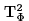that we met in the previous section. We’ll learn that one can define a Berry connection over the Brillouin zone and that the associated Chern number determines the Hall conductivity.

TKNN Invariants
We’ll consider a particle moving on a rectangular lattice. The distance between lattice sites in the x-direction is a; the distance in the y-direction is b. Recall from earlier courses that the energy spectrum of this system form bands. Within each band, states are labelled by lattice momentum which takes values in the Brillouin zone, parame-terised by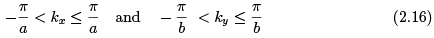The states with momenta at the edges of the Brillouin zone are identified. This means that the Brillouin zone is a torus T2 as promised. The wavefunctions in a given band can be written in Bloch form as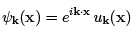(2.7)
where uk(x) is usually periodic on a unit cell so that uk(x + e) = uk(x) with either e = (a, 0) or e = (0, b).
We’re now in a position to describe the topology underlying the quantum Hall effect. The results below are very general: they don’t rely on any specific Hamiltonian, but rather apply to any system that satisfies a few simple criteria.

• First, we will assume that the single particle spectrum decomposes into bands, with each band parameterised by a momentum label k which lives on a torus T2. This is obviously true for simple lattice models. As we explain in Section
• It is also true (under certain assumptions) for particles moving in a lattice in the presence of a magnetic field where the torus in question is slightly different concept called a magnetic Brillouin zone. (In this case, the periodicity conditions on uk are altered slightly but the formula we derive below still holds.)
• Second, we’ll assume that the electrons are non-interacting. This means that we get the multi-particle spectrum simply by filling up the single-particle spectrum, subject to the Pauli exclusion principle.
• Finally, we’ll assume that there is a gap between bands and that the Fermi energy Ef lies in one of these gaps. This means that all bands below Ef are completely filled while all bands above Ef are empty. In band theory, such a situation describes an insulator.

Whenever these three criteria are obeyed, one can assign an integer-valued topological invariant C ∈ Z to each band. The topology arises from the way the phase of the states winds as we move around the Brillouin zone T2. This is captured by a U(1) Berry connection over T2, defined by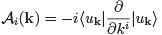There is one slight conceptual difference from the type of Berry connection we met previously. In Section 1.5, the connections lived on the space of parameters of the Hamiltonian; here the connection lives on the space of states itself. Nonetheless, it is simple to see that many of the basic properties that we met in Section 1.5 still hold. In particular, a change of phase of the states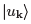corresponds to a change of gauge of the Berry connection.
We can compute the field strength associated to Ai. This is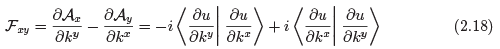Once again, we can compute the first Chern number by integrating F over the Brillouin zone T2,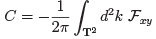(2.19)
In the present context, it is usually referred to as the TKNN invariant10. As we’ve seen before, the Chern number is always an integer: C ∈ Z. In this way, we can associate an integer Cα to each band α.
The Chern number once again has a beautiful physical manifestation: it is related to the Hall conductivity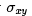of a non-interacting band insulator by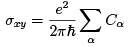(2.20)
where the sum is over all filled bands α and Cα is the Chern class associated to that band. This is the famous TKNN formula. It is, of course, the same formula (2.15) that we met previously, although the context here is rather different.
Let’s now prove the TKNN formula. Our starting point is the Kubo formula (2.12). We previously wrote this in terms of multi-particle wavefunctions. If we’re dealing with non-interacting particles, then these can be written as tensor products of single particle wavefunctions, each of which is labelled by the band a and momentum k ∈ T2 The expression for the Hall conductivity becomes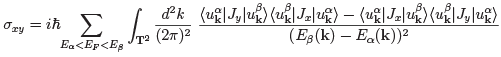where the index a runs over the filled bands and β runs over the unfilled bands. We note that this notation is a little lazy; there are really separate momentum integrals for each band and no reason that the states in the expression have the same momentum k. Our lazy notation will save us from adding yet more annoying indices and not affect the result below.
To make progress, we need to define what we mean by the current J. For a single, free particle in the continuum, the current carried by the particle was simply J = ex where the velocity operator is x = (p + eA)/m. Here we’ll use a more general definition. We first look at the Schrodinger equation acting on single-particle wavefunctions of Bloch form (2.17),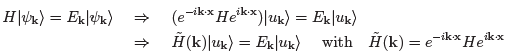We then define the current in terms of the group velocity of the wavepackets,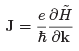Before proceeding, it’s worth checking that coincides with our previous definition. In the continuum, the Hamiltonian was simply H = (p + eA)2/2m, which gives H = (p + ℏk + eA)2/2m and the current due to a single particle is J = ex as expected.
From now on it’s merely a question of doing the algebra. The Kubo formula becomes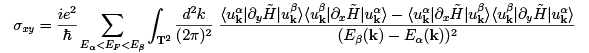where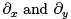in this expression are derivatives with respect to momenta kx and ky respectively. We can then write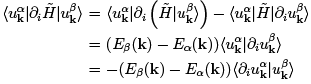The missing term, proportional to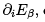, doesn’t appear because α and β are necessarily distinct bands. Substituting this into the Kubo formula gives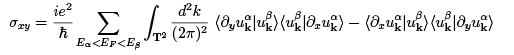But now we can think of the sum over the unfilled bands as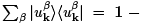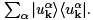The second term vanishes by symmetry, so we’re left with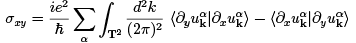where now the sum is only over the filled bands a. Comparing to (2.18), we see that the Hall conductivity is indeed given by the sum of the Chern numbers of filled bands as promised,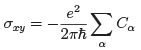The TKNN formula is the statement that the Hall conductivity is a topological invariant of the system. It’s important because it goes some way to explaining the robustness of the integer quantum Hall effect. An integer, such as the Chern number C, can’t change continuously. This means that if we deform our system in some way then, as long as we retain the assumptions that went into the derivation above, the Hall conductivity can’t change: it’s pinned at the integer value.
The existence of the TKNN formula is somewhat surprising. The right-hand side is simple and pure. In contrast, conductivities are usually thought of as something complicated and messy, depending on all the intricate details of a system. The essence of the TKNN formula, and indeed the quantum Hall effect itself, is that this is not the case: the Hall conductivity is topological.

The Chern Insulator
Let’s look at an example. Perhaps surprisingly, the simplest examples of lattice models with non-vanishing Chern numbers don’t involve any magnetic fields at all. Such lattice models with filled bands are sometimes called Chern insulators, to highlight the fact that they do something interesting — like give a Hall response — even though they are insulating states.
The simplest class of Chern insulators involve just two bands. The single-particle Hamiltonian written, written in momentum space, takes the general form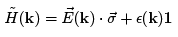where k ∈ T2 and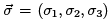are the three Pauli matrices. The energies of the two states with momentum k are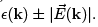. An insulator requires a gap between the upper and lower bands; we then fill the states of the lower band. An insulator can only occur when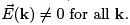For any such model, we can introduce a unit three-vector,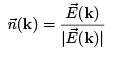Clearly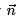describes a point on a two-dimensional sphere S2. This is the Bloch sphere. As we move in the Brillouin zone,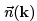gives a map from T2 → S2 as shown in the figure. This Chern number (2.19) for this system can be written in terms of  asas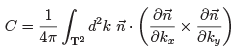There is a particularly nice interpretation of this formula: it measures the area of the unit sphere (counted with sign) swept out as we vary k over T2. In other words, it counts how many times T2 wraps around S2.
Perhaps the simplest lattice model with a non-trivial Chern number arises on a square lattice, with the Hamiltonian in momentum space given by11.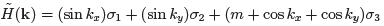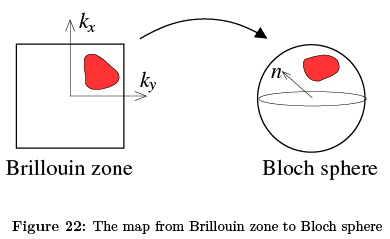In the continuum limit, this becomes the Hamiltonian for a 2-component Dirac fermion in d = 2 + 1 dimensions. For this reason, this model is sometimes referred to as a Dirac-Chern insulator.
For general values of m, the system is an insulator with a gap between the bands. There are three exceptions: the gap closes and the two bands touch at m = 0 and at m = ±2. As m varies, the Chern number — and hence the Hall conductivity — remains constant as long as the gap doesn’t close. A direct computation gives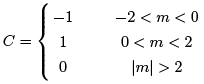Particles on a Lattice in a Magnetic Field
So far, we’ve discussed the integer quantum Hall effect in lattice models but, perhaps surprisingly, we haven’t explicitly introduced magnetic fields. In this section, we describe what happens when particles hop on a lattice in the presence of a magnetic field. As we will see, the physics is remarkably rich.
To orient ourselves, first consider a particle hopping on two-dimensional square lattice in the absence of a magnetic field. We’ll denote the distance between adjacent lattice sites as a. We’ll work in the tight-binding approximation, which means that the position eigenstates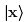are restricted to the lattice sites x = a(m, n) with m, n ∈ Z. The Hamiltonian is given by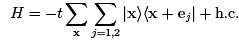(2.21)
where e1 = (a, 0) and e2 = (0, a) are the basis vectors of the lattice and t is the hopping parameter. (Note: t is the standard name for this parameter; it’s not to be confused with time!) The lattice momenta k lie in the Brillouin zone T2, parameterised by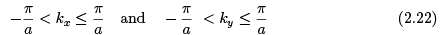Suppose that we further make the lattice finite in spatial extent, with size Ll x L2. The momenta ki are now quantised in units of 2π/Li. The total number of states in the Brillouin zone is then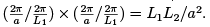. This is the number of sites in the lattice which is indeed the expected number of states in the Hilbert space.
Let's now add a background magnetic field to the story. The first thing we need to do is alter the Hamiltonian. The way to do this is to introduce a gauge field Ai (x) which lives on the links between the lattice sites. We take A1(x) to be the gauge field on the link to the right of point x, and A2(x) to be the gauge field on the link above point x, as shown in the figure. The Hamiltonian is then given by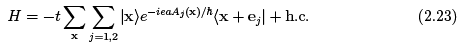It might not be obvious that this is the correct way to incorporate a magnetic field. To gain some intuition, consider a particle which moves anti-clockwise around a plaquette. To leading order in t, it will pick up a phase e-iY, where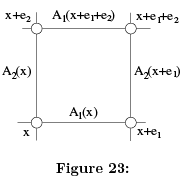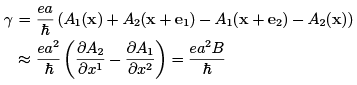where B is the magnetic field which passes through the plaquette. This expression is the same as the Aharonov-Bohm phase (1.49) for a particle moving around a flux Φ = Ba2.
Let’s now restrict to a constant magnetic field. We can again work in Landau gauge,
A1 = 0 and A2 = Bx1 (2.24)
We want to understand the spectrum of the Hamiltonian (2.23) in this case and, in particular, what becomes of the Brillouin zone.

Magnetic Brillouin Zone
We saw above that the key to finding topology in a lattice system was the presence of the Brillouin zone T2. Yet it’s not immediately obvious that the Brilliouin zone survives in the presence of the magnetic field. The existence of lattice momenta k are a consequence of the discrete translational invariance of the lattice. But, as usual, the choice of gauge breaks the explicit translational invariance of the Hamiltonian, even though we expect the underlying physics to be translational invariant.
In fact, we’ll see that the interplay between lattice effects and magnetic effects leads to some rather surprising physics that is extraordinarily sensitive to the flux Φ = Ba2 that threads each plaquette. In particular, we can define a magnetic version of the Brillouin zone whenever Φ is a rational multiple of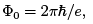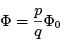(2.25)
with p and q integers which share no common divisor. We will see that in this situation the spectrum splits up into q different bands. Meanwhile, if Φ/Φ0 is irrational, there are no distinct bands in the spectrum: instead it takes the form of a Cantor set! Nonetheless, as we vary Φ/Φ0, the spectrum changes continuously. Needless to say, all of this is rather odd!
We start by defining the gauge invariant translation operators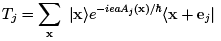This shifts each state by one lattice site; T1 moves us to the left and Ti to the right, while T2 moves us down and T2 up, each time picking up the appropriate phase from the gauge field. Clearly we can write the Hamiltonian as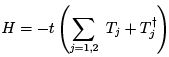These translation operators do not commute. Instead it's simple to check that they obey the nice algebra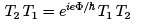(2.26)
This is the discrete version of the magnetic translation algebra (2.14). In the present context it means that [Ti, H] = 0 so, in the presence of a magnetic field, we don’t get to label states by the naive lattice momenta which would be related to eigenvalues of Ti. This shouldn’t be too surprising: the algebra (2.26) is a reflection of the fact that the gauge invariant momenta don’t commute in a magnetic field, as we saw in (1.15).
However, we can construct closely related operators that do commute with Tj and, hence, with the Hamiltonian. These are defined by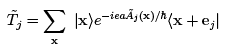where the new gauge field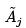is constructed to obey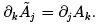. In Landau gauge, this means that we should take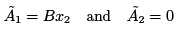When this holds, we have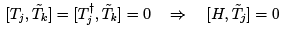These operators commute with the Hamiltonian, but do not themselves commute. Instead, they too obey the algebra (2.26).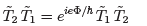(2.27)
This means that we could label states of the Hamiltonian by eigenvalues of, say,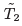but not simultaneously by eigenvalues of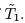This isn’t enough to construct a Brillouin zone.
At this point, we can see that something special happens when the flux is a rational multiple of Φ0, as in (2.25). We can now build commuting operators by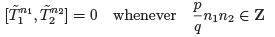This means in particular that we can label energy eigenstates by their eigenvalue underand, simultaneously, their eigenvalue under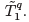. We call these states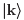with k = (k1,k2). They are Bloch-like eigenstates, satisfying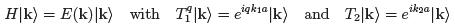Note that the momenta ki are again periodic, but now with the range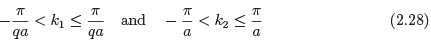The momenta ki parameterise the magnetic Brillouin zone. It is again a torus T2, but q times smaller than the original Brillouin zone (2.22). Correspondingly, if the lattice has size L1 x L2, the number of states in each magnetic Brillouin zone is L1L2/qa2. This suggests that the spectrum decomposes into q bands, each with a different range of energies. For generic values of p and q, this is correct.
The algebraic structure above also tells us that any energy eigenvalue in a given band is q-fold degenerate. To see this, consider the state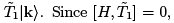, we know that this state has the same energy as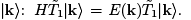But, using (2.27), the ky eigenvalue of this state is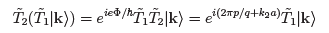We learn that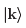has the same energy as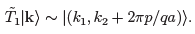The existence of a Brillouin zone (2.28) is the main result we need to discuss Hall conductivities in this model. However, given that we’ve come so far it seems silly not to carry on and describe what the spectrum of the Hamiltonian (2.23) looks like. Be warned, however, that the following subsection is a slight detour from our main goal.

To further understand the spectrum of the Hamiltonian (2.23), we’ll have to roll up our sleeves and work directly with the Schrodinger equation. Let’s first look in position space. We can write the most general wavefunction as a linear combination of the position eigenstates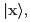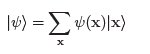The Schrodinger equation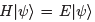then becomes an in nite system of coupled, discrete equations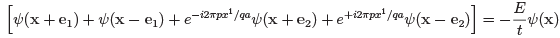We want to find the possible energy eigenvalues E.
The way we usually solve these kinds of problems is by doing a Fourier transform of the wavefunction to work in momentum space, with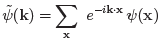(2.29)
where, since x takes values on a discrete lattice, k takes values in the original Brillouin zone (2.22). In the absence of a magnetic field, modes with different momenta k decouple from each other. However, if you try the same thing in the presence of a magnetic field, you’ll find that the modes with momentum k = (k1,k2) couple to modes with momentum (k+ 2πp/qa, k2). The reflects the fact that, as we have seen, the magnetic Brillouin zone (2.28) is q times smaller. For this reason, we instead split the wavefunction (2.29) into q different wavefunctions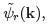, with r = 1,..., q as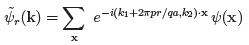These contain the same information as (2.29), but now the argument k ranges over the magnetic Brillouin zone (2.28). Given the wavefunctions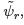we can always reconstruct by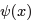the inverse Fourier transform,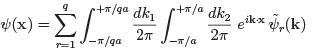In this way, we see that we have a q-component vector of wavefunctions,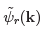living on the magnetic Brillouin zone.
Taking the Fourier transform of the discrete Schrodinger equation in position space yields the following equation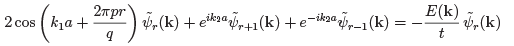This is known as the Harper equation.
The Harper equation can be solved numerically. The resulting spectrum is quite wonderful. For rational values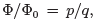, the spectrum indeed decomposes into q bands with gaps between them, as we anticipated above. Yet the spectrum also varies smoothly as we change Φ. Obviously if we change Φ/Φ0 continuously it will pass through irrational values; when this happens the spectrum forms something like a Cantor set. The result is a beautiful fractal structure called the Hofstadter butterfly12 shown in Figure 24. Here, a point is drawn in black if there is a state with that energy. Otherwise it is white. To get a sense of the structure, you could look at the specific values  Φ/Φ0 = 1/q, above which you should see q vertical bands of black.

TKNN Invariants for Particles on a Lattice in a Magnetic Field
Finally we reach our main goal: to compute the Hall conductivity of the lattice model for a particle in a background magnetic field. We can only do this for rational fluxes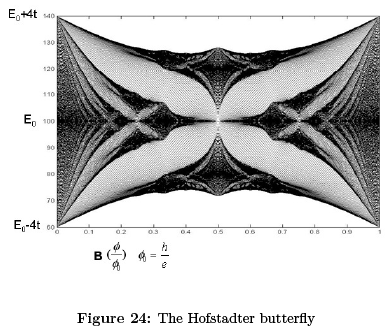Φ= pΦ0/q for which there exists a magnetic Brillouin zone. In this case, we can use the TKNN formula (2.20), but with the Chern number, which used to be defined by integrating over the Brillouin zone, now arising by integrating over the magnetic Brillouin zone.
The computation of the Chern numbers is not so straightforward. (You can find it in the original paper of TKNN or, in more detail, in the book by Fradkin). Here we just state the answer. Even this is not totally straightforward.
First consider the rth of the q bands. Then, to compute the Chern number, you are invited to solve the linear Diophantine equation
r = qsr + pt
with |tr| ≤ q/2. The Chern number of the rth band is given by
Cr = tr-tr-1
where t0 = 0. If the first r bands are filled, so that Er < EF < Er+1, then the Hall conductivity is given by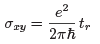It’s helpful to look at some examples. First, when Φ= pΦ0, there is only a single band and the Hall conductivity vanishes. A more complicated, illustrative example is given by p/q = 11/7. Here the solutions to the Diophantine equation are (sr ,tr) = (-3, 2), (5, -3), (2, -1), (-1,1), (-4, 3), (4, -2), (1, 0). As we fill consecutive bands, the second number tr in these pairs determines the Hall conductivity. We see that the Hall conductivity varies in an interesting way, sometimes negative and sometimes positive.

Offer running on EduRev: Apply code STAYHOME200 to get INR 200 off on our premium plan EduRev Infinity!

,

,

,

,

,

,

,

,

,

,

,

,

,

,

,

,

,

,

,

,

,

;